# ggHoriPlot

This vignette goes through all the functionality of the package. If you want to see examples with real data, you can refer to `vignette('examples', 'ggHoriPlot')`.

# Introduction

The data used through this vignette are tables with sine waves, which aims to mimic time-series data. The data looks like this:

``````library(tidyverse)
library(patchwork)
library(ggthemes)``````
``````x = 1:300
y = x * sin(0.1 * x)
dat_tab <- tibble(x = x,
xend = x+0.9999,
y = y)

x = 1:400
y = x * sin(0.2 * x) + 100
dat_tab_bis <- tibble(x = x,
xend = x+0.9999,
y = y)

tab_tot <- mutate(dat_tab, type = 'A') %>%
bind_rows(mutate(dat_tab_bis, type='B'))

tab_tot %>%
ggplot() +
geom_line(aes(x, y)) +
facet_wrap(~type, scales = 'free_y', ncol = 1) +
theme_few()``````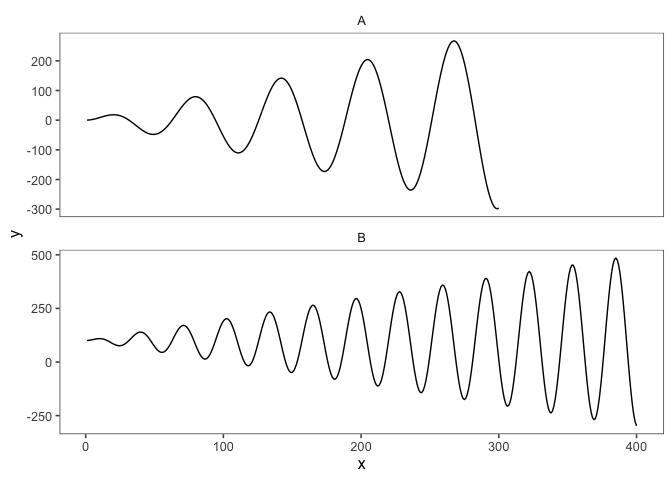This representation of the dataset is fine if we only have a few waves. However, if we aim to represent and compare time series with many entries, it might be challenging to plot them as line charts. A more convenient way to plot this type of datasets are horizon plots, which are able to condense the data but still retain all the information. You can learn more about horizon plots here.

`ggHoriPlot` allows you to easily build horizon plots in `ggplot2`. First we will load the package and a helper functions that can be used to visualize and compare horizon plots and line charts.

``````library(ggHoriPlot)

plotAllLayers <- function(dat, ori, cutpoints, colors){
# Helper function to plot the origin and cutpoints
# of the horizon plot for comparison
p <- ggplot()
acc <- 1
for (i in cutpoints[cutpoints<=ori]) {
colo <- colors[acc]
p <- p + geom_ribbon(aes(x = x, y = y, ymin = y, ymax = ori),
fill = colo,
data = mutate(dat, y = ifelse(between(y, i, ori), y,
ifelse(y<ori, i, ori))))
acc <- acc+1
}
for (i in cutpoints[cutpoints>=ori]) {
colo <- colors[acc]
p <- p + geom_ribbon(aes(x = x, y = y, ymin = ori, ymax = y),
fill = colo,
data = mutate(dat, y = ifelse(between(y, ori, i), y,
ifelse(y>ori, i, ori))))
acc <- acc+1
}

p+geom_line(aes(x, y), data=dat)+
theme_few()
}``````

We are now all set! By using `geom_horizon()` we can add a layer in the `ggplot2` framework to build a horizon plot.

``````a <- dat_tab %>%
ggplot() +
geom_horizon(aes(x = x, y=y))

a``````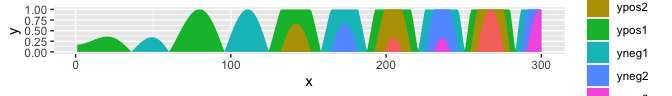The default `ggplot2` fill colors might not be the best choice of palette for horizon plots. Instead, we can use the `scale_fill_hcl()` function to choose an appropriate color scheme. The default palette will color low values red and large values blue.

``````a <- dat_tab %>%
ggplot() +
geom_horizon(aes(x = x, y=y)) +
theme_few()  +
scale_fill_hcl()

a``````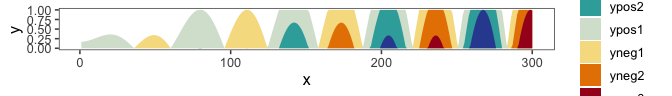To understand how horizon plots are related to line charts, we can plot both side by side.

``````a <- dat_tab %>%
ggplot() +
geom_horizon(
aes(x = x,
y=y)
) +
theme_few()  +
scale_fill_hcl()

cutpoints  <- tibble(
cuts = c(78.48221,  172.74027,  266.99833, -110.03390, -204.29196, -298.55001),
names = c('ypos1', 'ypos2', 'ypos3', 'yneg1', 'yneg2', 'yneg3'),
color = c("#D7E2D4", "#36ABA9", "#324DA0", "#F6DE90", "#E78200", "#A51122")
) %>%
mutate(names = factor(names, rev(names))) %>%
arrange(names)

mid <- sum(range(dat_tab\$y, na.rm = T))/2

b <- plotAllLayers(dat_tab, mid, cutpoints\$cuts, cutpoints\$color)

b/a+ plot_layout(guides = 'collect', heights = c(6, 1))``````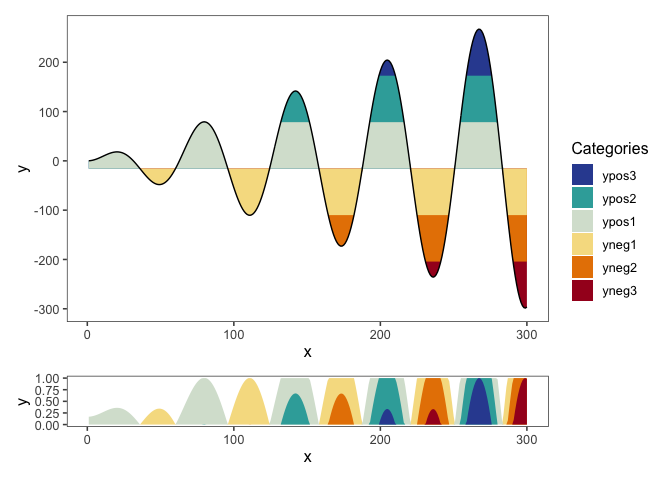The resulting figure shows how the sine curve of this example can be condensed into a stripe instead of a full line chart.

`ggHoriPlot` can also output the exact intervals for each cutpoint by simply adding `fill=..Cutpoints..` in the aesthetics:

``````a <- dat_tab %>%
ggplot() +
geom_horizon(
aes(x = x,
y=y,
fill=..Cutpoints..)
) +
theme_few()  +
scale_fill_hcl()

cutpoints  <- tibble(
cuts = c(78.48221,  172.74027,  266.99833, -110.03390, -204.29196, -298.55001),
names = c('ypos1', 'ypos2', 'ypos3', 'yneg1', 'yneg2', 'yneg3'),
color = c("#D7E2D4", "#36ABA9", "#324DA0", "#F6DE90", "#E78200", "#A51122")
) %>%
mutate(names = factor(names, rev(names))) %>%
arrange(names)

mid <- sum(range(dat_tab\$y, na.rm = T))/2

b <- plotAllLayers(dat_tab, mid, cutpoints\$cuts, cutpoints\$color)

b/a+ plot_layout(guides = 'collect', heights = c(6, 1))``````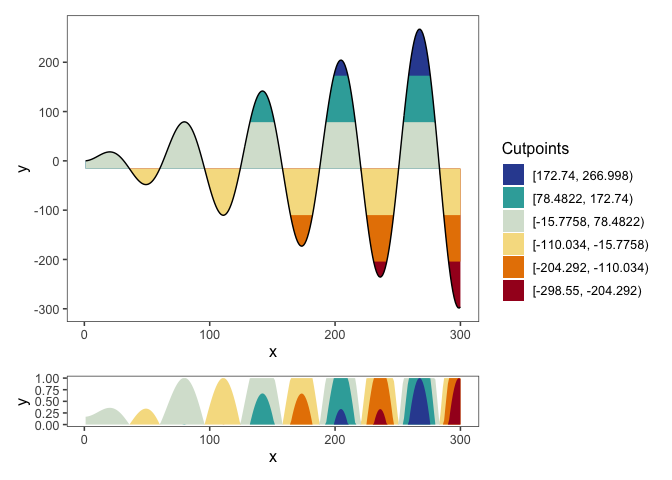# Changing the origin

## Median

The above example with default settings calculates the origin of the horizon plot as the midpoint between the data range. Sometimes, however, we might want to use some other origin for our data. In `ggHoriPlot` this can be achieved by specifying the desired `origin` argument in `geom_horizon()`. For example, if we want to use the median as the origin:

``````a <- dat_tab %>%
ggplot() +
geom_horizon(
aes(x = x,
y=y,
fill=..Cutpoints..),
origin = 'median'
) +
theme_few() +
scale_fill_hcl()

cutpoints  <- tibble(
cuts = c(96.20134, 190.4594, 284.7174, -92.31478, -186.57283, -280.83089),
names = c('ypos1', 'ypos2', 'ypos3', 'yneg1', 'yneg2', 'yneg3'),
color = c("#D7E2D4", "#36ABA9", "#324DA0", "#F6DE90", "#E78200", "#A51122")
) %>%
mutate(names = factor(names, rev(names))) %>%
arrange(names)

me <- median(dat_tab\$y, na.rm = T)

b <- plotAllLayers(dat_tab, me, cutpoints\$cuts, cutpoints\$color)

b/a+ plot_layout(guides = 'collect', heights = c(6, 1))``````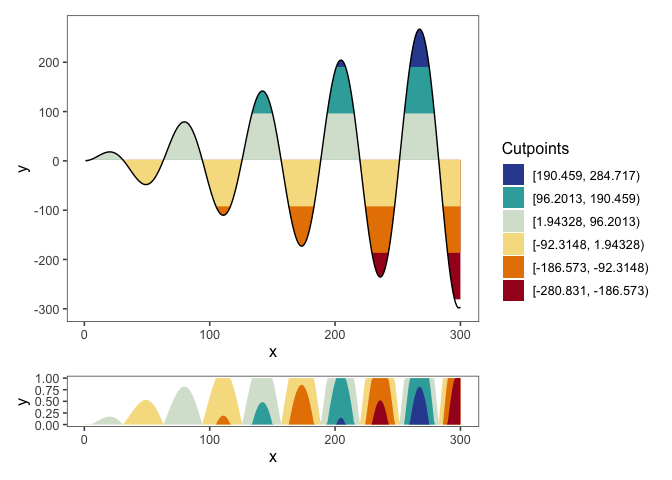Note that the horizon scale –this is, the regular interval that determines the cutpoints–, is still the same as when using the midpoint. This might produce some cutpoints that do not entirely match the range of values. In the above example, limit for the upper interval (the bluest interval) falls outside of the range of values. At the other end, the limit for the lower interval (the reddest interval) falls within the range of the data. All the data values that are above the upper limit or (as happens in this case) below the lower limit are colored as the closest interval.

## Mean

The origin can also be specified to be the mean:

``````a <- dat_tab %>%
ggplot() +
geom_horizon(
aes(x = x,
y=y,
fill=..Cutpoints..),
origin = 'mean'
) +
theme_few() +
scale_fill_hcl()

cutpoints  <- tibble(
cuts = c(91.89319,  186.15125,  280.40931,  -96.62292, -190.88098, -285.13903),
names = c('ypos1', 'ypos2', 'ypos3', 'yneg1', 'yneg2', 'yneg3'),
color = c("#D7E2D4", "#36ABA9", "#324DA0", "#F6DE90", "#E78200", "#A51122")
) %>%
mutate(
names = factor(names, rev(names)),
y_max = ifelse(cuts == min(cuts),
-Inf,
ifelse(
cuts == max(cuts),
Inf,
cuts))) %>%
arrange(names)

me <- mean(dat_tab\$y, na.rm = T)

b <- plotAllLayers(dat_tab, me, cutpoints\$cuts, cutpoints\$color)

b/a+ plot_layout(guides = 'collect', heights = c(6, 1))``````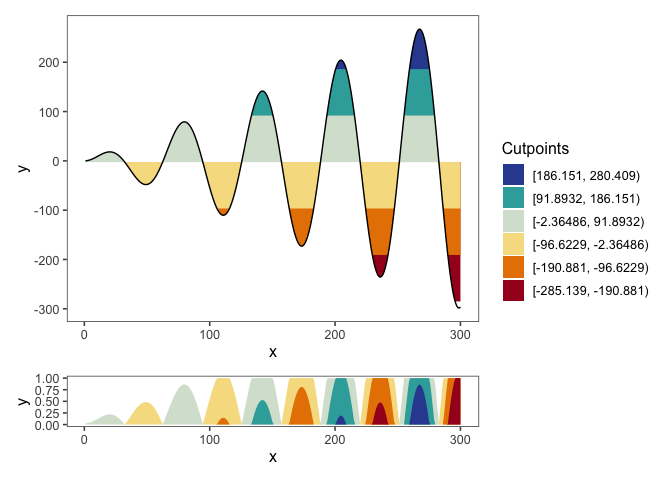## User-specified

Alternatively, the origin might also be a manually chosen number:

``````a <- dat_tab %>%
ggplot() +
geom_horizon(
aes(x = x,
y=y,
fill=..Cutpoints..),
origin = 50
) +
theme_few() +
scale_fill_hcl()

cutpoints  <- tibble(
cuts = c(144.25806,  238.51611,  332.77417,  -44.25806, -138.51611, -232.77417),
names = c('ypos1', 'ypos2', 'ypos3', 'yneg1', 'yneg2', 'yneg3'),
color = c("#D7E2D4", "#36ABA9", "#324DA0", "#F6DE90", "#E78200", "#A51122")
) %>%
mutate(names = factor(names, rev(names))) %>%
arrange(names)

b <- plotAllLayers(dat_tab, 50, cutpoints\$cuts, cutpoints\$color)

b/a+ plot_layout(guides = 'collect', heights = c(6, 1))``````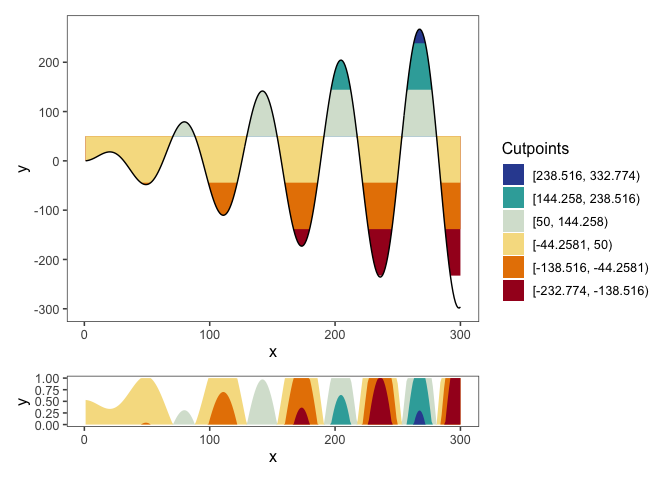## Quantiles

If we specify the origin to be `quantiles`, then the origin will be set to the median and the cutpoints will be set to equally sized quantiles:

``````a <- dat_tab %>%
ggplot() +
geom_horizon(
aes(x = x,
y=y,
fill=..Cutpoints..),
origin = 'quantiles'
) +
theme_few() +
scale_fill_hcl()

cutpoints  <- tibble(
cuts = c(43.02642,  124.15063,  266.99833,  -45.43396, -119.31147, -298.55001 ),
names = c('ypos1', 'ypos2', 'ypos3', 'yneg1', 'yneg2', 'yneg3'),
color = c("#D7E2D4", "#36ABA9", "#324DA0", "#F6DE90", "#E78200", "#A51122")
) %>%
mutate(names = factor(names, rev(names))) %>%
arrange(names)

me <- median(dat_tab\$y, na.rm = T)

b <- plotAllLayers(dat_tab, me, cutpoints\$cuts, cutpoints\$color)

b/a+ plot_layout(guides = 'collect', heights = c(6, 1))``````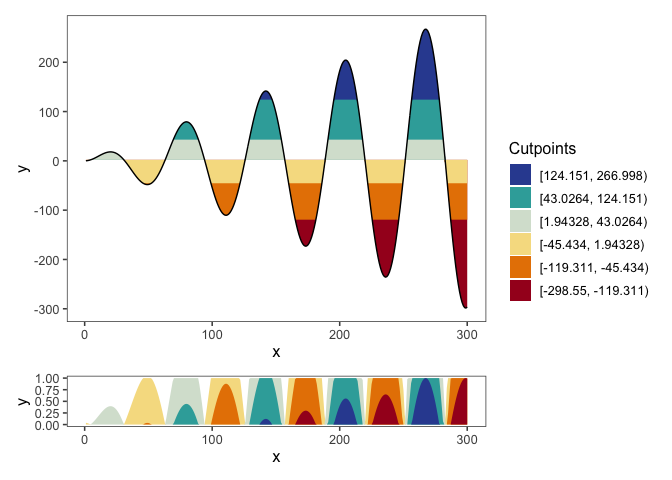Note that this might produce intervals that do not have the same size, which can be undesirable and/or deceiving.

## Origin as lowest value

Sometimes we are not interested in plotting values as both above and below the origin. In those cases, we can specify the origin to be the smallest value by setting `origin='min'`, so all values are above the origin.

``````a <- dat_tab %>%
ggplot() +
geom_horizon(
aes(x = x,
# xend = xend,
y=y,
fill=..Cutpoints..),
horizonscale = 6,
origin = 'min'
) +
theme_few() +
scale_fill_hcl()

cutpoints_a  <- tibble(
cuts = c(-15.78, 78.48,  172.74,  266.998, -110.034, -204.292, -298.55),
color = c("#D7E2D4", "#36ABA9", "#324DA0", 'white', "#F6DE90", "#E78200", "#A51122")
)

cutpoints_a  <- cutpoints_a %>% arrange(desc(cuts))

b <- plotAllLayers(dat_tab, -298.55, cutpoints_a\$cuts, cutpoints_a\$color)

(b/a) + plot_layout(guides = 'collect', heights = c(6, 1))``````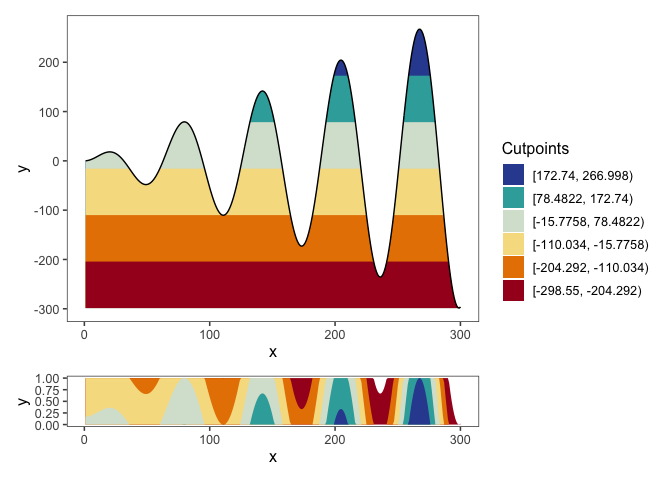For this example the red and blue coloring does not make much sense. Instead, you can choose another hcl palette and specify it in `scale_fill_hcl()`. For example, a single-hue palette is much more appropriate:

``````a <- dat_tab %>%
ggplot() +
geom_horizon(
aes(x = x,
# xend = xend,
y=y,
fill=..Cutpoints..),
horizonscale = 6,
origin = 'min'
) +
theme_few() +
scale_fill_hcl(palette = 'Purple-Orange', reverse = T)

cutpoints_a  <- tibble(
cuts = c(-15.78, 78.48,  172.74,  266.998, -110.034, -204.292, -298.55),
color = c( "#B76AA8", "#8F4D9F","#5B3794", 'white', "#D78CB1", "#F1B1BE", "#F8DCD9")
)

cutpoints_a  <- cutpoints_a %>% arrange(desc(cuts))

b <- plotAllLayers(dat_tab, -298.55, cutpoints_a\$cuts, cutpoints_a\$color)

(b/a) + plot_layout(guides = 'collect', heights = c(6, 1))``````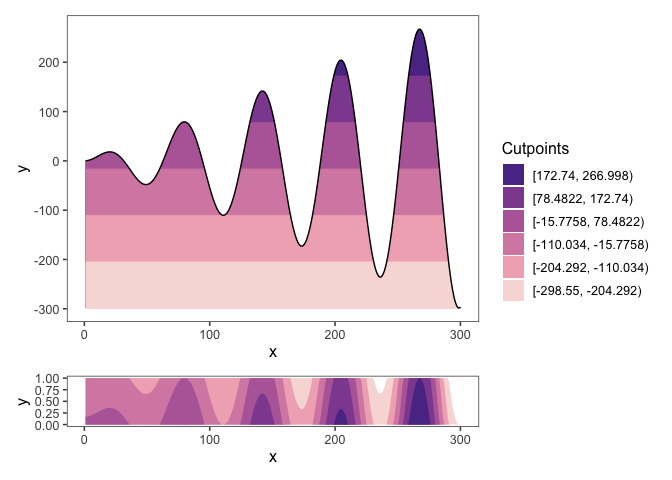You can list all available palettes by running `hcl.pals()`.

# Changing the horizon scale

Apart from the origin, `ggHoriPlot` also allows to customize the horizon scale, this is, the number of cuts and where they happen. The default number of cuts is set to 6, as in all of the examples above, but it can be set to any other integer, such as 5 intervals:

``````a <- dat_tab %>%
ggplot() +
geom_horizon(
aes(x = x,
# xend = xend,
y=y,
fill=..Cutpoints..),
horizonscale = 5,
origin = 'midpoint'
) +
theme_few() +
scale_fill_hcl()

cutpoints  <- tibble(
cuts = c(97.33383,  210.44349, -128.88551, -241.99518, -355.10485),
names = c('ypos1', 'ypos2',  'yneg1', 'yneg2', 'yneg3'),
color = c("#69BBAB", "#324DA0", "#FEFDBE", "#EB9C00", "#A51122")
) %>%
mutate(names = factor(names, rev(names))) %>%
arrange(names)

mid <- sum(range(dat_tab\$y, na.rm = T))/2

b <- plotAllLayers(dat_tab, mid, cutpoints\$cuts, cutpoints\$color)

b/a+ plot_layout(guides = 'collect', heights = c(5, 1))``````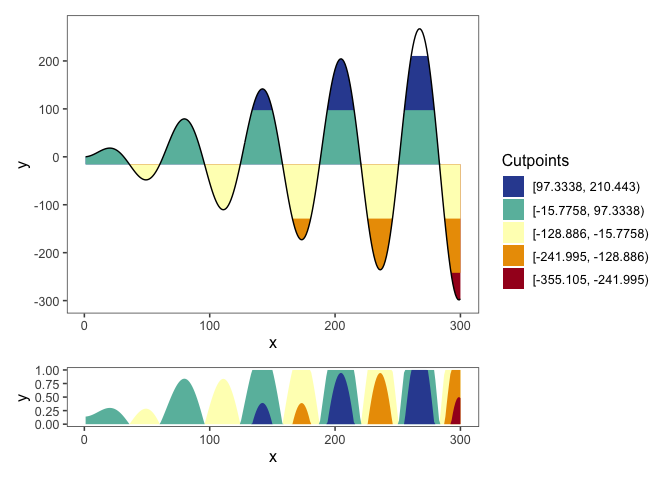``````a <- dat_tab %>%
ggplot() +
geom_horizon(
aes(x = x,
# xend = xend,
y=y,
fill=..Cutpoints..),
horizonscale = 10,
origin = 'midpoint'
) +
theme_few() +
scale_fill_hcl()

cutpoints  <- tibble(
cuts = c(40.77899 ,  97.33383 , 153.88866,  210.44349 , 266.99833 ,
-72.33068, -128.88551, -185.44035, -241.99518, -298.55001),
names = c('ypos1', 'ypos2', 'ypos3', 'ypos4', 'ypos5', 'yneg1', 'yneg2', 'yneg3', 'yneg4', 'yneg5'),
color = c("#E5F0D6", "#ACD2BB" ,"#4EB2A9" ,"#0088A7", "#324DA0",
"#FAEDA9","#F1C363","#E98E00", "#DC4A00", "#A51122")
) %>%
mutate(names = factor(names, rev(names))) %>%
arrange(names)

mid <- sum(range(dat_tab\$y, na.rm = T))/2

b <- plotAllLayers(dat_tab, mid, cutpoints\$cuts, cutpoints\$color)

b/a + plot_layout(guides = 'collect', heights = c(10, 1))``````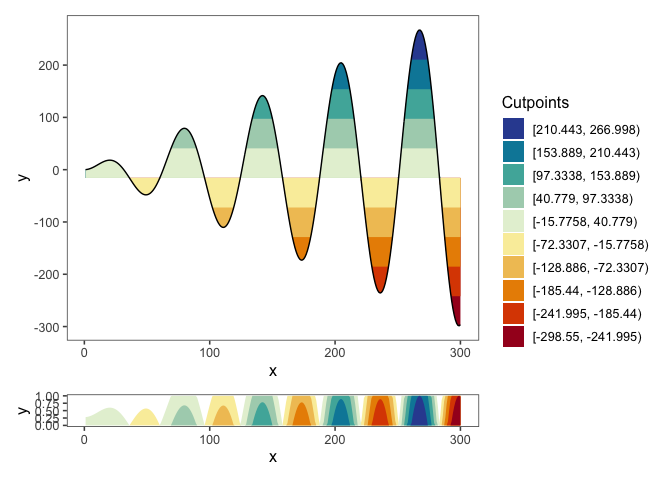Finally, we can also specify our own intervals by providing a vector of cutpoints:

``````a <- dat_tab %>%
ggplot() +
geom_horizon(
aes(x = x,
y=y,
fill=..Cutpoints..),
horizonscale = c(78.48221,  172.74027,
266.99833, -110.03390,
-204.29196, -298.55001),
origin = -15.77584
) +
theme_few()  +
scale_fill_hcl()

cutpoints  <- tibble(
cuts = c(78.48221,  172.74027,  266.99833, -110.03390, -204.29196, -298.55001),
names = c('ypos1', 'ypos2', 'ypos3', 'yneg1', 'yneg2', 'yneg3'),
color = c("#D7E2D4", "#36ABA9", "#324DA0", "#F6DE90", "#E78200", "#A51122")
) %>%
mutate(names = factor(names, rev(names))) %>%
arrange(names)

mid <- sum(range(dat_tab\$y, na.rm = T))/2

b <- plotAllLayers(dat_tab, mid, cutpoints\$cuts, cutpoints\$color)

b/a+ plot_layout(guides = 'collect', heights = c(6, 1))``````## Interval data

Some data might have starting and end points for x values. If that is the case, the line chart will have a step-like shape. `ggHoriPlot` can also plot this kind of data. We simply need to specify the end coordinates using the `xend` aesthetics inside `geom_horizon()`:

``````a <- dat_tab %>%
ggplot() +
geom_horizon(
aes(x = x,
xend = xend,
y=y,
fill=..Cutpoints..),
horizonscale = 6,
origin = 'midpoint'
) +
theme_few() +
scale_fill_hcl()

cutpoints  <- tibble(
cuts = c(78.48221,  172.74027,  266.99833, -110.03390, -204.29196, -298.55001),
names = c('ypos1', 'ypos2', 'ypos3', 'yneg1', 'yneg2', 'yneg3'),
color = c("#D7E2D4", "#36ABA9", "#324DA0", "#F6DE90", "#E78200", "#A51122")
) %>%
mutate(names = factor(names, rev(names))) %>%
arrange(names)

dt <- dat_tab %>%
pivot_longer(c(x, xend)) %>%
mutate(x = value)

b <- plotAllLayers(dt, mid, cutpoints\$cuts, cutpoints\$color)

b/a+ plot_layout(guides = 'collect', heights = c(6, 1))``````